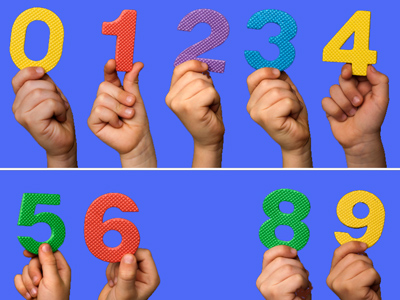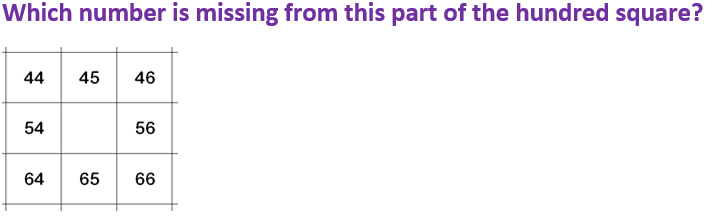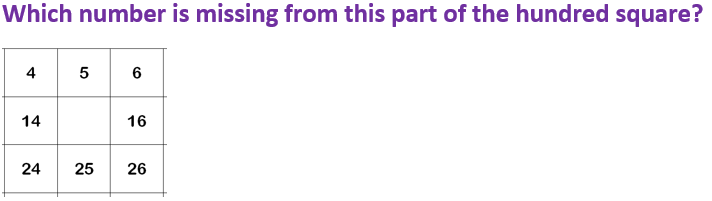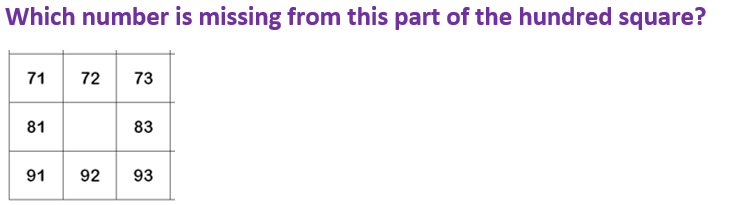Being able to recognize the missing number in a sequence is vitally important in math.

# Place Value Problem Solving

This Math quiz is called 'Place Value Problem Solving' and it has been written by teachers to help you if you are studying the subject at elementary school. Playing educational quizzes is a fun way to learn if you are in the 1st or 2nd grade - aged 6 to 8.

It costs only \$12.50 per month to play this quiz and over 3,500 others that help you with your school work. You can subscribe on the page at Join Us

In elementary school children begin to learn about what each digit in a number represents, or its place value. For example, in the number 34, there are 3 tens and 4 units. They can use this knowledge of place values to solve problems using 100 squares, answer more than/less than questions, solve money problems and also use it to answer number bond questions.

Can you solve the place value problems?

Question 155
85
59
32
55 is one more than 54, and 10 more than 45
Question 26
15
23
27
15 is one less than 16 and ten more than 5
Question 3
Kate has 42p. Joe has 10p more. How much does Joe have?
62p
52p
32p
22p
10p more means adding one 10 in the tens column
Question 4
Which number is 20 less than 80?
60
67
63
70
’20 less’ means counting back 20 from 80, or subtracting 20
Question 5
Which number is missing from this number sentence?
12 + ? = 20
8
18
12
10
Knowing numbers bonds or pairs to 20 helps here
Question 685
82
38
88
82 is one less than 83, and ten less than 92
Question 7
Which number is 4 more than 65?
66
69
79
80
‘4 more’ means adding 4 to 65
Question 8
I have £1 and spend 50p. How much do I have left?
80p
20p
60p
50p
£1 is 100p. 50p less than this is 50p
Question 9
Which number is missing from this number sentence?
20 - ? = 13
17
23
7
13
13 + 7 = 20, so 20 – 7 must be 13!
Question 10
Which number is 10 more than 43?
44
54
63
53
‘10 more’ means adding 10 to the number
Author:  Angela Smith